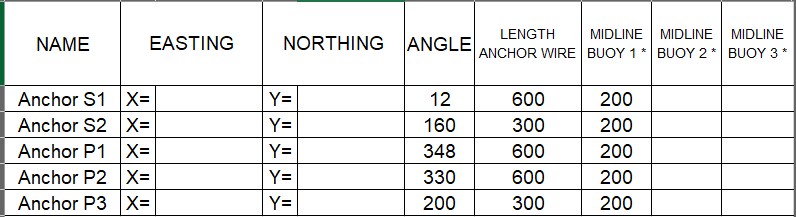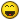# How to sort list with a letter-number elements

## Recommended PostsHi,

I want to sort a list based on the following  elements:

Input: ((S1 (x y z)) (P2 (x y z)) (S2 (x y z)) (P1 (x y z)) (P3 (x y z)) (S3 (x y z)))

Result Output: ((S1 (x y z)) (S2 (x y z)) (S3 (x y z)) (P1 (x y z)) (P2 (x y z)) (P3 (x y z)))

So element (S1 (x y z)) needs to be the first element of the list, followed by all other S number elements in ascending order.
after the S number elements the P number elements must be sorted the same way.

Also the list does not contain a fixed amount of S and P elements, so the number sequence for each letter may vary

Anyone an idea how to do this?

##### Share on other sites3 hours ago, gsc said:

Input: ((S1 (x y z)) (P2 (x y z)) (S2 (x y z)) (P1 (x y z)) (P3 (x y z)) (S3 (x y z)))

Result Output: ((S1 (x y z)) (S2 (x y z)) (S3 (x y z)) (P1 (x y z)) (P2 (x y z)) (P3 (x y z)))

just dummy idea not tested, but if letter ascending should be P=first S=after

`(mapcar 'read (acad_strlsort (mapcar 'vl-princ-to-string lst)))`

##### Share on other sitesThe vl-sort supports multi level sorting so can have say multi S1's and then second sort on X Y Z. This should make the P occur 1st.

```; sorts on 1st two items
(vl-sort lst
'(lambda (a b)
(cond
((< (car a) (car b)))
)
)
)

;x only
(setq lst (vl-sort lst '(lambda (x y) (< (car x)(car y)))))

(vl-sort '(3 2 1 20 98.32 16 0 20 -2.345) '<)
(-2.345 0 1 2 3 16 20 98.32) only 1 20

(setq test '(87 6.54 20 1 8.88 20))   ; contains two 20's
(mapcar 'cdr (vl-sort (mapcar '(lambda (x) (cons 1 x)) test) '(lambda (y z) (< (cdr y) (cdr z)))))
returns:
(1 6.54 8.88 20 20 87); both 20's still there```

##### Share on other sitesPosted (edited)

I know but S stands for Starboard Side and P for Port Side.
The S and P elements are (hidden) attributes in a block (which is a ship) representing an anchor location at the ship.
S1 and P1 for instance are Tag Names and also the default Values of the attribute objects.
The ship (in block mode) is always pointing to the North with the 0,0 origin at the stern (not necessarily in the middle)

With a sub routine, the WCS coordinates of the anchors are stored in a LIST for later use.

The insertion point of the attributes is actually the start point of an anchor line which is drawn

However the List order must be according to this table, which is used as an input to read in (the known) angle, length anchor wire data and possible mid line buoy distance.

But the table is also placed into the drawing, filled with the (calculated) anchor coordinates after setting them out with the (relative) angle and distance (length anchor wire).

Each ship/barge we use may have a different amount of Starboard Side and Port Side anchors, so S1 and P1 are always there, but the amount (= the number) may vary per ship/barge.
Now it would be easier if I changed the order (P1 first row) in the excel sheet, but my internal clients are a bit autistic...they want to see the S anchors first in the tableFor now I have changed the default values of the hidden attributes to A1 (for Starboard) and B1 (for Port), because this way the function of hanhphuc works.

But it would be nicer if the result list could be manipulated to the correct order:

`(mapcar 'read (acad_strlsort (mapcar 'vl-princ-to-string lst)))`

Result Output: ((P1 (x y z)) (P2 (x y z)) (P3 (x y z)) (S1 (x y z)) (S2 (x y z)) (S3 (x y z)))

Desired output: ((S1 (x y z)) (S2 (x y z)) (S3 (x y z)) (P1 (x y z)) (P2 (x y z)) (P3 (x y z)))

So after the first code split the result list between P and S elements and shift all S elements left to first position.
Is that possible?

Edited by gsc

##### Share on other sites1 hour ago, gsc said:

For now I have changed the default values of the hidden attributes to A1 (for Starboard) and B1 (for Port), because this way the function of hanhphuc works.

But it would be nicer if the result list could be manipulated to the correct order:

```

Result Output: ((P1 (x y z)) (P2 (x y z)) (P3 (x y z)) (S1 (x y z)) (S2 (x y z)) (S3 (x y z)))

Desired output: ((S1 (x y z)) (S2 (x y z)) (S3 (x y z)) (P1 (x y z)) (P2 (x y z)) (P3 (x y z)))

So after the first code split the result list between P and S elements and shift all S elements left to first position.
Is that possible?

`if your list pattern is simple, vl-string-translate `
`(mapcar '(lambda(x) (read (vl-string-translate "PS" "SP" x))) (acad_strlsort (mapcar 'vl-princ-to-string lst)))`

FWIW..  sorting string is way different

(acad_strlsort  '("P3" "P1" "P2" "P123" "P11"))

; ("P1" "P11" "P123" "P2" "P3")

numeric sort (vl-sort '(1 11 123 2 3) '<)

;'(1 2 3 11 123)

##### Share on other sitesAn easier way may be look at P or S then add to correct list Plst or Slst sort both of them individually then join them into final lst the desired output. Note below the sort is greater than saves a reverse list

```(setq lstp (list  (list "P2" (list 4 4 6))  (list "P1" (list 1 2 3)) (list "P3" (list 0 1 2 ))))
(setq lsts (list (list "S1" (list 1 2 3)) (list "S3" (list 4 5 6)) (list "S2" (list 7 8 9))))
(setq lstp (vl-sort lstp '(lambda (x y) (> (car x)(car y)))))
(setq lsts (vl-sort lsts '(lambda (x y) (> (car x)(car y)))))
(setq lst '())
(foreach x lstp
(setq lst (cons x lst))
)
(foreach x lsts
(setq lst (cons x lst))
)```

##### Share on other sitesPfff....still struggling with this sub routine. Let me be more specific.

The goal of this sub routine is to iterate through all present attributes in a block, and list their value and wcs coordinate.

```(defun getatt ( blk / enx )
(if (and (setq blk (entnext blk)) (= "ATTRIB" (cdr (assoc 0 (setq enx (entget blk))))))
(setq lst
(cons
(cons
(cdr (assoc 1 enx)) ; attrib value
(cdr (assoc 10 enx)) ; wcs coordinate
)
(getatt blk)
)
)
)
)```

The result list is:

`((S2 239.209 265.528 0.0) (P1 259.56 341.311 0.0) (P2 256.249 342.843 0.0) (S1 271.666 335.713 0.0) (P3 223.721 272.691 0.0))`

I deliberately created the attributes in a dis-order because users who will create these attribute blocks may do the same.

But the list should be ordered like this for later use:

`((S1 271.666 335.713 0.0) (S2 239.209 265.528 0.0) (P1 259.56 341.311 0.0) (P2 256.249 342.843 0.0) (P3 223.721 272.691 0.0))`

S items first followed by P items, because I want the coordinates to be listed in a certain order
The amount of S and P items may vary per attribute block

How do I do this efficiently?

##### Share on other sitesPosted (edited)

If lst contains your list then

`(setq lst (vl-sort lst '(lambda (x y) (if (= (substr (car x) 1 1) (substr (car y) 1 1)) (< (atoi (substr (car x) 2)) (atoi (substr (car y) 2))) (> (substr (car x) 1 1) (substr (car y) 1 1))))))`

As S1, S2 ..P# are strings (attribute tags) and the (cadr) of each item in the list is itself a list (coordinates)

Edited by dlanorh

##### Share on other sitesWow that was quick!...this is working...thanx man

##### Share on other sites32 minutes ago, gsc said:

Wow that was quick!

Nope. It was a late reply to your first post.##### Share on other sitesNice dlanorh maybe atof rather than atoi as example has decimals.

## Join the conversation

You can post now and register later. If you have an account, sign in now to post with your account.
Note: Your post will require moderator approval before it will be visible.×   Pasted as rich text.   Paste as plain text instead

Only 75 emoji are allowed.